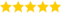# Convert Decimal To Binary In Python

Last updated on 25th Sep 2020, Artciles, Blog

Ramanan (Sr Technical Project Manager )

He is a TOP-Rated Domain Expert with 6+ Years Of Experience, Also He is a Respective Technical Recruiter for Past 3 Years & Share's this Informative Articles For Freshers(5.0) | 16547 Ratings 797

In this post, we will see programs to convert decimal numbers to an equivalent binary number. We will see two Python programs, the first program does the conversion using a user defined function and in the second program we are using a in-built function bin() for the decimal to binary conversion.

### 1. Decimal to Binary conversion using recursive function

In this program we have defined a function decimalToBinary() for the conversion. This function takes the decimal number as an input parameter and converts it into an equivalent binary number.

• def decimalToBinary(num):
• “””This function converts decimal number
• to binary and prints it”””
• if num > 1:
• decimal To Binary(num // 2)
• print(num % 2, end=”)
• # decimal number
• number = int(input(“Enter any decimal number: “))
• decimalToBinary(number)

### 1. To convert the floating point into decimal, we have 3 elements in a 32-bit floating point representation:

i) Sign

ii) Exponent

iii) Mantissa

• Sign bit is the first bit of the binary representation. ‘1’ implies negative number and ‘0’ implies positive numbers.
Example: 11000001110100000000000000000000 This is negative number.
• Exponent is decided by the next 8 bits of binary representation. 127 is the unique number for 32 bit floating point representation. It is known as bias. It is determined by 2k-1 -1 where ‘k’ is the number of bits in the exponent field.
There are 3 exponent bits in 8-bit representation and 8 exponent bits in 32-bit representation.
Thus
bias = 3 for 8 bit conversion (23-1 -1 = 4-1 = 3)
bias = 127 for 32 bit conversion. (28-1 -1 = 128-1 = 127)

### Python Program to Convert the given number from Decimal to Binary

In this article, let us discuss how to convert the given input number from decimal to binary using two different methods.

To find a binary equivalent, the decimal number has to be recursively divided by the value 2 until the decimal number reaches the value zero. After each and every division, the remainder has to be noted. The reverse of the remainder values gives the binary equivalent of the decimal number.

For example, the binary conversion of the decimal number 15 is shown in the below image.

### INPUT FORMAT:

Input consists of an integer

### OUTPUT FORMAT:

Binary equivalent of the given input decimal number

14

1110

### Algorithm for converting Decimal to Binary

Step 1: Get an input value from the user

Step 2: If the input is greater than zero, divide the number by the value 2 and note the remainder

Step 3: Repeat Step 2 until the decimal number reaches zero

Step 4: Print the remainder values

Step 5: End

### Method1: Using user-defined function

Python program to convert the given decimal number to binary using a user-defined function

• def decimalToBinary(num):if num > 1:
• decimal To Binary(num // 2)
• print(num % 2, end = ”)
• number = int(input(“Enter the decimal number: “))
• #main() function
• decimalToBinary(number)

### Input:

Enter the decimal number: 25

11001

### PREREQUISITE KNOWLEDGE:

Built-In Functions in Python

### Method 2: Using built-in function

When we use the built-in function, the output will be obtained with the prefix ‘ob’.

Python program to convert the given decimal number to binary using a built-in function

• number = int(input(“Enter the decimal number: “))
• print(bin(number))

### Input:

Enter the decimal number: 56

### Output:

Binary Number: 0b111000

Are you looking training with Right Jobs?